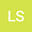Ground state solution for a periodic p\&q-Laplacain equation involving critical growth without the Ambrosetti-Rabinowitz condition
•## Abstract

We study the ground state solutions for the following p\&q-Laplacain equation $\left\{ \begin{array}{ll} -\Delta_pu-\Delta_qu+V(x) (|u|^{p-2}u+|u|^{q-2}u)=\lambda K(x)f(u)+|u|^{q^*-2}u,~x\in\R^N, \\ u\in W^{1,p}(\R^N)\cap W^{1,q}(\R^N), \end{array} \right.$ where $\lambda>0$ is a parameter large enough, $\Delta_ru = \text{div}(|\nabla u|^{r-2}\nabla u)$ with $r\in\{p,q\}$ denotes the $r$ Laplacian operator, \$1
14 Aug 2021Submitted to Mathematical Methods in the Applied Sciences
14 Aug 2021Submission Checks Completed
14 Aug 2021Assigned to Editor
20 Nov 2022Reviewer(s) Assigned
27 Jan 2023Review(s) Completed, Editorial Evaluation Pending
27 Jan 2023Editorial Decision: Revise Minor